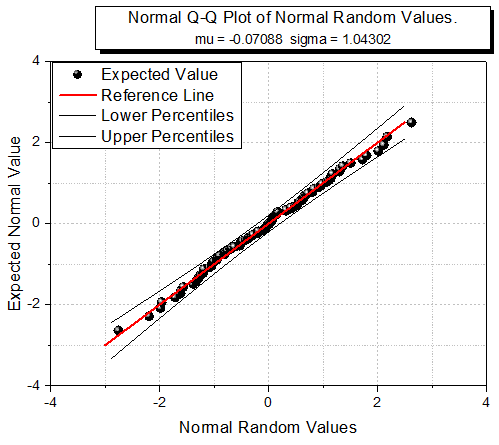Skip Navigation Links.

Q-Q PlotLearn how to create this graph here.

Description:

A Q-Q plot is a graphical method for testing whether a dataset follows a given distribution. In a Q-Q plot, observed values are plotted on the X-axis and expected values are plotted on the Y-axis. If all scatter points are close to the reference line, we can say that the dataset follows the given distribution.

Origin supports five given distributions (Normal, Lognormal, Exponential, Weibull and Gamma), and provides five plotting methods for approximating percentiles (Blom, Benard, Hazen, Var der Waerden, and Kaplan-Meier).

In this Q-Q plot, we have selected Normal Distribution and the Benard Score Method. Note that Origin supports to output the confidence band in the Q-Q plot by selecting the Confidence Band check box since Origin 2016.